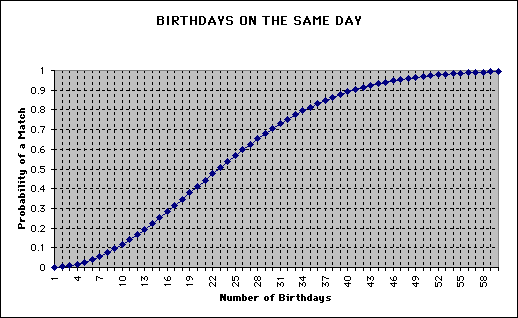College of Education

# Office for Mathematics, Science, and Technology Education

Resources Projects Contact
Home Activity Birthday Explanation

# The Birthday Problem - explanation

Let's look at the probabilities a step at a time.

• For one person, there are 365 distinct birthdays.
• For two people, there are 364 different ways that the second could have a birthday without matching the first.
• If there is no match after two people, the third person has 363 different birthdays that do not match the other two. So, the probability of a match is 1 - (365)(364)(363)/(365)(365)(365).
• This leads to the following formula for calculating the probability of a match with N birthdays is 1 - (365)(364)(363)...(365 - N + 1)/(365)^N.

Running this through a computer gives the chart below. Notice that a probability of over .5 is obtained after 23 dates!Notice that the probability is above .9
before the sample size reaches even 45.